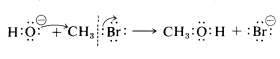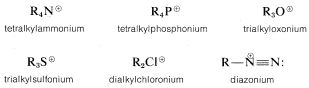# 8.4: General Considerations of Substitution Reactions

$$\newcommand{\vecs}{\overset { \rightharpoonup} {\mathbf{#1}} }$$ $$\newcommand{\vecd}{\overset{-\!-\!\rightharpoonup}{\vphantom{a}\smash {#1}}}$$$$\newcommand{\id}{\mathrm{id}}$$ $$\newcommand{\Span}{\mathrm{span}}$$ $$\newcommand{\kernel}{\mathrm{null}\,}$$ $$\newcommand{\range}{\mathrm{range}\,}$$ $$\newcommand{\RealPart}{\mathrm{Re}}$$ $$\newcommand{\ImaginaryPart}{\mathrm{Im}}$$ $$\newcommand{\Argument}{\mathrm{Arg}}$$ $$\newcommand{\norm}{\| #1 \|}$$ $$\newcommand{\inner}{\langle #1, #2 \rangle}$$ $$\newcommand{\Span}{\mathrm{span}}$$ $$\newcommand{\id}{\mathrm{id}}$$ $$\newcommand{\Span}{\mathrm{span}}$$ $$\newcommand{\kernel}{\mathrm{null}\,}$$ $$\newcommand{\range}{\mathrm{range}\,}$$ $$\newcommand{\RealPart}{\mathrm{Re}}$$ $$\newcommand{\ImaginaryPart}{\mathrm{Im}}$$ $$\newcommand{\Argument}{\mathrm{Arg}}$$ $$\newcommand{\norm}{\| #1 \|}$$ $$\newcommand{\inner}{\langle #1, #2 \rangle}$$ $$\newcommand{\Span}{\mathrm{span}}$$$$\newcommand{\AA}{\unicode[.8,0]{x212B}}$$

We now wish to discuss displacements by nucleophilic reagents ($$Y:$$) on alkyl derivatives ($$RX$$). These are ionic or polar reactions involving attack by a nucleophile at carbon. A typical example is the reaction of hydroxide ion with bromomethane to displace bromide ion:The electron pair of the $$C-O$$ bond can be regarded as having been donated by the hydroxide ion, while the electron pair of the $$C-Br$$ bond departs with the leaving bromide ion. The name for this type of reaction is abbreviated $$\text{S}_\text{N}$$, $$\text{S}$$ for substitution and $$\text{N}$$ for nucleophilic.

Reactions of this type are very useful. They can lead to compounds in which the new bond to carbon in the alkyl group, $$R$$ is to chlorine, bromine, iodine, oxygen, sulfur, carbon, nitrogen, or phosphorus, depending on the nature of the nucleophile used.

Nucleophilic substitutions are especially important for alkyl halides, but they should not be considered to be confined to alkyl halides. Many other alkyl derivatives such as alcohols, ethers, esters, and "onium ions"$$^3$$ also can undergo $$\text{S}_\text{N}$$ reactions if conditions are appropriate. The scope of $$\text{S}_\text{N}$$ reactions is so broad that it is impossible to include all the various alkyl compounds and nucleophiles that react in this manner. Rather we shall approach the subject here through consideration of the mechanisms of $$\text{S}_\text{N}$$ reactions, and then develop the scope of the reactions in later chapters.

The mechanism of an $$\text{S}_\text{N}$$ reaction and the reactivity of a given alkyl compound $$RX$$ toward a nucleophile $$Y$$ depend upon the nature of $$R$$, $$X$$, and $$Y$$, and upon the nature of the solvent. For an $$\text{S}_\text{N}$$ reaction to occur at a reasonable rate, it is very important to select a solvent that will dissolve both the alkyl compound and the nucleophilic reagent; considerable assistance may be required from both the solvent and the nucleophile to break what usually is a slightly polar $$C-X$$ bond. However, the solvents that best dissolve slightly polar organic compounds seldom will dissolve the common, rather highly polar, nucleophilic agents such as $$NaBr$$, $$NaCN$$, and $$H_2O$$. In practice, relatively polar solvents, or solvent mixtures, such as 2-propanone (acetone), aqueous 2-propanone, ethanol, aqueous 1,4-dioxacyclohexane (dioxane), and so on, provide the best compromise for reactions between alkyl compounds and saltlike nucleophilic reagents. The importance of the solvent in stabilizing ions can be evaluated from the estimated thermochemistry of ionic reactions discussed in Section 8-2.

$$^3$$Examples of -onium cations are